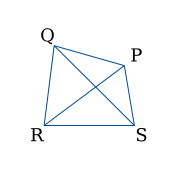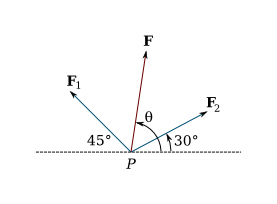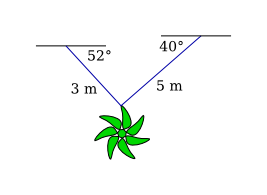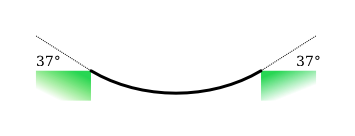Vectors

(requires JavaScript)

1. Write each combination of directed line segments as a single directed line segment.
1. $\stackrel{\to }{PQ}+\stackrel{\to }{QR}$
2. $\stackrel{\to }{RP}+\stackrel{\to }{PS}$
3. $\stackrel{\to }{QS}-\stackrel{\to }{PS}$
4. $\stackrel{\to }{RS}+\stackrel{\to }{SP}+\stackrel{\to }{PQ}$2. Given $A\left(2,3\right)$ and $B\left(-2,1\right)$, find a vector $\mathbf{a}$ with representation given by the directed line segment $\stackrel{\to }{AB}$. Draw $\stackrel{\to }{AB}$ and the equivalent representation starting at the origin.
$\mathbf{a}=⟨-4,-2⟩$
3. Find the sum of vectors $⟨0,1,2⟩$ and $⟨0,0,-3⟩$ and illustrate geometrically.
$⟨0,1,-1⟩$
4. Find $\mathbf{a}+\mathbf{b}$, $2\mathbf{a}+3\mathbf{b}$, $\left|\mathbf{a}\right|$, and $\left|\mathbf{a}-\mathbf{b}\right|$, where $\mathbf{a}=2\mathbf{i}-4\mathbf{j}+4\mathbf{k}$ and $\mathbf{b}=2\mathbf{j}-\mathbf{k}$.
5. Find a unit vector with the same direction as $8\mathbf{i}-\mathbf{j}+4\mathbf{k}$.
$\frac{8}{9}\mathbf{i}-\frac{1}{9}\mathbf{j}+\frac{4}{9}\mathbf{k}$
6. The two forces ${\mathbf{F}}_{1}$ and ${\mathbf{F}}_{2}$ with magnitudes $10$ lb and $12$ lb respectively, act on an object at a point $P$ as shown in the figure. Find the resultant force $\mathbf{F}$ acting at $P$ as well as its magnitude and its direction. (Indicate the direction by finding the angle $\theta$ shown in the figure.)$\mathbf{F}=\left(6\sqrt{3}-5\sqrt{2}\right)\mathbf{i}+\left(6+5\sqrt{2}\right)\mathbf{j}$,
$\left|\mathbf{F}\right|\approx 13.5$ lb,
$\theta \approx 76°$.
7. Velocities have both direction and magnitude and thus are represented by vectors. The magnitude of a velocity is called speed. Suppose that a wind is blowing from the direction N$45°$W at a speed of $50$ km/h. (This means that the direction from which the wind blows is $45°$ west of northerly direction.) A pilot is steering a helicopter in the direction N$60°$E at an airspeed (speed in still air) of $80$ km/h. The true course, or track, of an aircraft is the direction of the resultant of the velocity vectors of the aircraft and the wind. The ground speed of the aircraft is the magnitude of the resultant. Find the true course and the ground speed of the helicopter.
8. Chains $3$ and $5$ m in length are fastened to a suspended decoration. The decoration has a mass of $5$ kg. The chains, fastened at different heights, make angles of $52°$ and $40°$ with the horizontal. Find the tension in each chain and the magnitude of each tension.9. The tension at each end of the rope has magnitude $25$ N. What is the weight of the rope?Let ${\mathbf{T}}_{1}$ and ${\mathbf{T}}_{2}$ be the tensions. By symmetry, their horizontal components cancel and their vertical components are equal. The magnitudes of vertical components of ${\mathbf{T}}_{1}$ and ${\mathbf{T}}_{2}$ add up to $2\left|{\mathbf{T}}_{1}\right|\mathrm{sin}\left(37°\right)$. Since the chain is at rest, the force ${\mathbf{T}}_{1}+{\mathbf{T}}_{2}$ must have the same magnitude as that of the force due to gravity, $\left|m\mathbf{g}\right|$, where $\left|\mathbf{g}\right|=9.8$. So we can write

$2\left|{\mathbf{T}}_{1}\right|\mathrm{sin}\left(37°\right)=9.8m$, and hence
$m=\frac{50\mathrm{sin}\left(37°\right)}{9.8}$,
$m\approx 3.07$ [kg].

10. Use vectors to prove that the line joining the midpoints of two sides of a triangle is parallel to the third side and half its length.